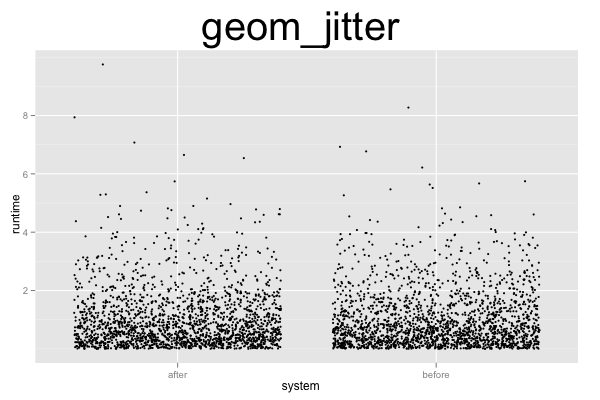## ggplot2 Quick Reference: geom_jitter

A geom that draws a point defined by an x and y coordinate, like geom_point, but jitters the points.

Default statistic: stat_identity

### Parameters

• x - (required) x coordinate of the point
• y - (required) y coordinate of the point
• size - (default: 0.5) diameter of the point
• shape - (default: 16=dot) the shape of the point
• colour - (default: "black") the color of the point
• fill - (default: NA) the fill of the point (only a small minority of shapes actually can be filled; see shape)
• alpha - (default: 1=opaque) the transparency of the point
• na.rm - (default: FALSE) silently remove points with NA coordinates

### Example

Jittering makes most sense when visualizing a large number of individual observations. In this example, we have a total of 4000 observations coming from two different systems. The discrete x axis represents the two systems. The continuous y axis represents the runtime. Without jittering, we would essentially see two vertical lines. With jittering, we can spread the points along the x axis (note that there also is some jittering along the y axis, but that amount is miniscule because `position_jitter` automatically determines the default amount of jitter based on the resolution of the data).```d=data.frame(system=rep(c('before','after'), 2000), runtime=rexp(4000, 1))
ggplot() +
geom_jitter(data=d, mapping=aes(x=system, y=runtime), size=1) +
opts(title="geom_jitter", plot.title=theme_text(size=40, vjust=1.5))```

### Note

To adjust the amount of jittering along the x and y axes, one can explicitly set the position adjustment:

`geom_jitter(data=d, mapping=aes(x=system, y=runtime), size=1, position=position_jitter(width=0.3, height=0)) `

Because the only difference between geom_jitter and geom_point is the automatically set position adjustment, the `geom_point(...)` layer below is identical to the `geom_jitter(...)` layer above.

`geom_point(data=d, mapping=aes(x=system, y=runtime), size=1, position=position_jitter(width=0.3, height=0)) `